# R语言估计多元标记的潜过程混合效应模型（lcmm）分析心理测试的认知过程

• X(t) 和 Z(t) 是协变量的向量（Z(t) 包含在 X(t) 中；
• β是固定效应（即总体平均效应）；
• ui 是随机效应（即个体效应）；它们根据具有协方差矩阵 B 的零均值多元正态分布进行分布；
• (wi(t)) 是一个高斯过程。

# 背景和定义

• αik~N(0,σ2k)
• Xcijk协变量向量
• γk 是对比（k 上的总和等于 0）
• tijk 对象 i、标记 k 和场合 j 的测量时间；
• ϵijk一个独立的高斯误差，均值为 0，方差为 σ2ϵkσϵk2；
• Hk将潜过程转换为标记 k 的尺度和度量的链接函数（由 ηk 参数化）。

• 线性变换：这简化为线性混合模型（2 个参数）
• Beta 累积分布族重新调整（4 个参数）
• 具有 m 个节点的二次 I 样条的基（m+2 个参数）

# 认知过程示例

## 模型考虑：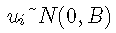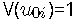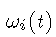是布朗过程，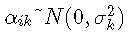对于 k = 1,2,3：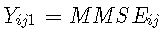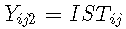和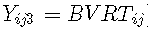# R语言如何用潜类别混合效应模型（lcmm）分析抑郁症状

## 不同链接函数的估计

```

tie <- (ae - ag_it)/10

ag75 <- (ae_it - 75)/10```

## 线性链接函数

`mlmm( ubc'ID', dt = pud, radom = T, cr =B(tme))`

## 非线性链接函数

`hist(MMSE)`

# 在单变量情况下，可以考虑 Beta CDF 或样条。链接函数族可以对所有标记都相同（即使参数不同）：

```

# 以Beta为例
mlmm( lnk = 'beta')```

# 请注意，样条变换有时可能涉及非常接近 0 的参数，从而导致无法收敛（因为参数位于参数空间的边界）。

```

# 样条曲线中不同数量的结点

mlmm( lik = c('eta','3-uan-spes','3-antpln'))```

## 修复一些转换参数

```

# 样条曲线

mlmm( axe=50, ink = c('3asin'))```

`best`

```

# 样条曲线

mult(B=mp\$best)```

## 模型比较

mult对象是多元潜在过程混合模型，它们假设潜过程的轨迹完全相同，但链接函数不同。在单变量情况下，可以使用信息标准来比较模型。该 `summary` 给我们这样的信息。

```

sumrtbe(ml)```

```

par(mrow=c(1,1))

plot(llnes2, col = c(ol\[2\],ol\[3\]ol4\]), ld =1,ly=4)```

## 估计的链接函数：

``` predict(ml_btapl)

plt(Cl)```

# 概括

`summary`

# 方差解释

```

VarE(tbsp,dtafme(tme=0))```

# 标记的预测轨迹图

```predct(btapl,nwdta=dtew,va.tim='ime')

plt(prec_we, ld=c(1)```

# 拟合优度：残差图

```

plt(mlep, 0.8)```

# 拟合优度：预测与观察的关系图

`plot(beal, whch="fit", time="ti")`

Kaizong Ye拓端研究室（TRL）的研究员。

​非常感谢您阅读本文，如需帮助请联系我们！QQ在线咨询

15121130882

0571-63341498

## 关注有关新文章的微信公众号

This will close in 0 seconds